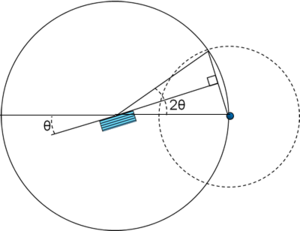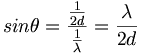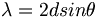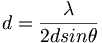# X-ray Diffraction

## Contents

### Background

X-ray diffraction (XRD) is a tool for characterizing arrangement of atoms in crystals and distances between crystal faces. This can be used to identify atoms and the crystalline form.

### Significance

X-ray diffraction is caused by constructive interference of x-ray waves that reflect off internal crystal planes. A thin film or layer of powder is fixed in the path of monochromatic x-rays. A detector measures x-rays from the sample over a range of angles. The powder consists of tiny crystals randomly oriented. At certain angles of the sensor populations of crystals have the correct angle so that Bragg's equation is satisfied for one of the crystal planes, resulting in a spike in X-rays. The output graph is x-ray intensity over 2 theta, the angle of the detector.Reflection (constructive interference) happens only where the wave path-length difference 2d sin θ equals an integer multiple of the wavelength λ.The XRD measures the diffraction angle 2 Theta with respect to the x-ray beam$sin \theta = \frac {\frac {1} {2d}} \frac {1} {\lambda} = \frac {\lambda} {2d }\,\!$$\lambda = 2d sin \theta\,\!$$d= \frac {\lambda} {2dsin\theta}\,\!$

X-ray diffraction interactive animation

### Operation

X-diffractometer for powder samples

Preparing a plate for identification of lead using XRD

X-diffractometer for thin films.